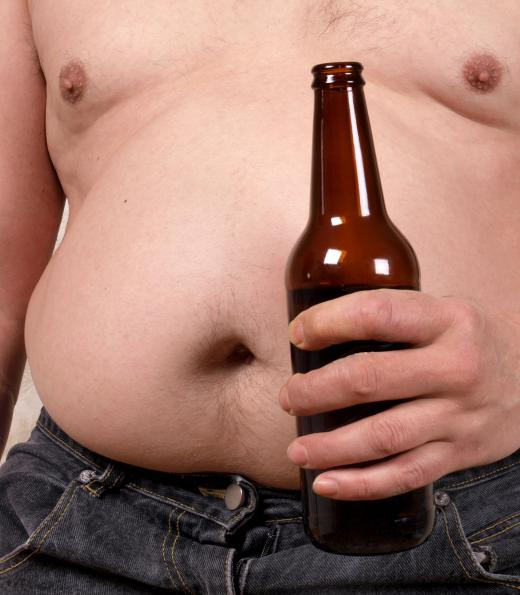Fitness
Fact Checked

# How do I Calculate Basal Metabolic Rate?

D. Messmer
D. Messmer

Knowing how you can calculate basal metabolic rate can be very helpful when trying to formulate an effective diet and exercise plan. The basal metabolic rate, or BMR, establishes the number of calories a person's body will burn in a single day if that person were to remain completely inactive throughout the day. Knowing how to calculate basal metabolic rate, then, can help a person know the quantities of calories that he or she needs to consume in order to gain or lose weight. BMR also can help a person know how many extra calories he or she should try to burn through exercise. It is not difficult to calculate basal metabolic rate, but the formula that men should use is different from the formula that women should use.

The calculations of BMR for men and women follow the same basic formula, but the fixed values in the formula are different. For men who use Imperial measurements, the formula is as follows: 66 + (12.7 x height in inches) + (6.23 x weight in pounds) - (6.8 x age). That formula for men using the metric system is as follows: 66 + (5 x height in centimeters) + (13.7 x weight in kilograms) - (6.8 x age). Women who use Imperial measurements should use the following formula: 655 + (4.7 x height in inches) + (4.35 x weight in pounds) - (4.7 x age). Using the metric system, women would use the following formula: 655 + (1.8 x height in centimeters) + (9.6 x weight in kilograms) - (4.7 x age).Calculating basal metabolic rate can be useful for formulating an effective diet and exercise plan for the purpose of gaining or losing weight.

The strength of the BMR formula is its ability to take many factors into account. Unlike many other health indexes, BMR considers more than just the subject's weight. Instead, it recognizes that as we age, our metabolism slows down, and it recognizes that men tend to have a higher rate of metabolism. BMR provides a clear indicator of resting metabolic rate, so taking these factors into account is crucial for an accurate measurement.Trying to calculate basal metabolic rate for the very overweight is often not useful.

There are, however, some limitations to overall accuracy when we calculate basal metabolic rate. BMR does not account for the amount of lean body mass and thus does not consider the ratio of lean body mass to total body mass. Thus, two people of the same gender, who are the same height, weight and age will always have the same BMR. If one of those people was overweight because of high body fat, and the other's weight was the result of being very muscular, though, their resting metabolic rate would be quite different. For this reason, trying to calculate basal metabolic rate for the very muscular or the very overweight is often not as useful.

## You might also Like